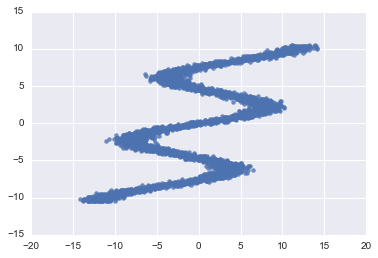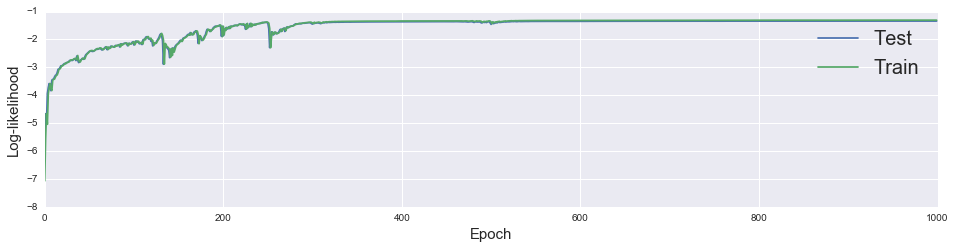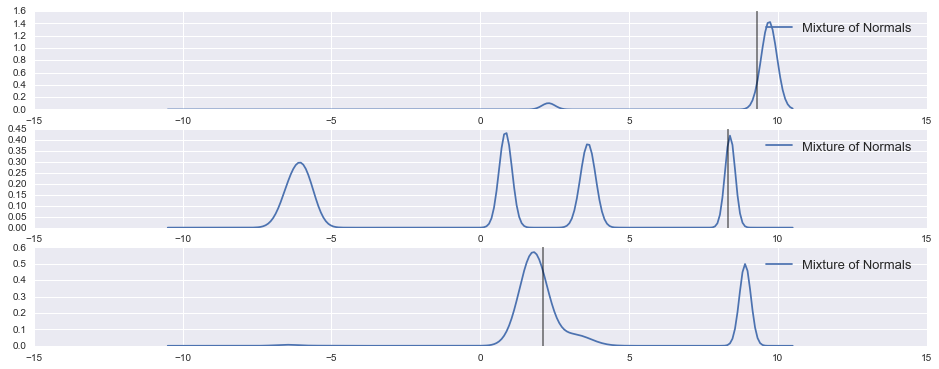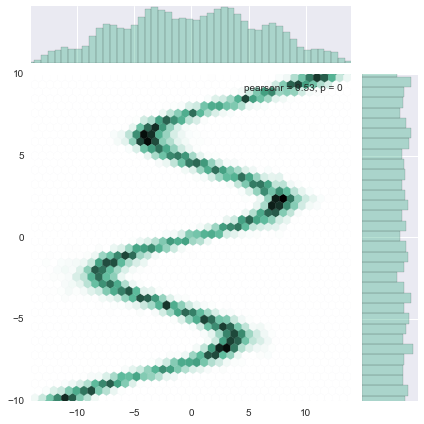# Mixture Density Networks with Edward, Keras and TensorFlow

In the previous blog post we looked at what a Mixture Density Network is with an implementation in TensorFlow. We then used this to learn the distance to galaxies on a simulated data set. In this blog post we'll show an easier way to code up an MDN by combining the power of three python libraries.

You are likely familiar with number 2 and 3 so let me tell you a bit about the first. Edward is a python library for probabilistic modelling, inference, and criticism. It's goal it to fuse the related areas of Bayesian Statistics, Machine Learning, Deep Learning and Probabilistic Programming. Edward is developed by the group of David Blei at Columbia University with the main developer being Dustin Tran. The example we discuss here is based on the example in the Edward repo that was written by Dustin and myself.

Edward implements many probability distribution functions that are TensorFlow compatible, this makes it attractive to use for MDN's. In the previous blog post we had to roll our own $Beta$ distribution, with Edward this is no longer necessary. Keep in mind, if you want to use Keras and TensorFlow like we will do in this post you need to set the backend of Keras to TensorFlow, here it is explained how to do that.

Here are all the distributions that are currently implemented in Edward, there are more to come:

Which all can be used to make a Mixture Density Networks. Let start by doing the imports.

In :
# imports
%matplotlib inline
import matplotlib.pyplot as plt
import seaborn as sns

import edward as ed
import numpy as np
import tensorflow as tf

from edward.stats import norm # normal distribution from Edward !
from keras import backend as K
from keras.layers import Dense
from sklearn.cross_validation import train_test_split


## Make some toy-data to play with.¶

This is the same toy-data problem set as used in the blog post by Otoro where he explains MDNs. This is an inverse problem as you can see, for every X there are multiple possible y solutions.

In :
def build_toy_dataset(nsample=40000):
y_data = np.float32(np.random.uniform(-10.5, 10.5, (1, nsample))).T
r_data = np.float32(np.random.normal(size=(nsample,1))) # random noise
x_data = np.float32(np.sin(0.75*y_data)*7.0+y_data*0.5+r_data*1.0)
return train_test_split(x_data, y_data, random_state=42, train_size=0.1)

X_train, X_test, y_train, y_test = build_toy_dataset()
print("Size of features in training data: {:s}".format(X_train.shape))
print("Size of output in training data: {:s}".format(y_train.shape))
print("Size of features in test data: {:s}".format(X_test.shape))
print("Size of output in test data: {:s}".format(y_test.shape))

sns.regplot(X_train, y_train, fit_reg=False)

Size of features in training data: (4000, 1)
Size of output in training data: (4000, 1)
Size of features in test data: (36000, 1)
Size of output in test data: (36000, 1)

Out:
<matplotlib.axes._subplots.AxesSubplot at 0x112680f90>## Building a MDN using Edward, Keras and TF¶

We will define a class that can be used to construct MDNs. In this notebook we will be using a mixture of Normal Distributions. The advantage of defining a class is that we can easily reuse this to build other MDNs with different amount of mixture components. Furthermore, this makes it play nice with Edward.

In :
class MixtureDensityNetwork:
"""
Mixture density network for outputs y on inputs x.
p((x,y), (z,theta))
= sum_{k=1}^K pi_k(x; theta) Normal(y; mu_k(x; theta), sigma_k(x; theta))
where pi, mu, sigma are the output of a neural network taking x
as input and with parameters theta. There are no latent variables
z, which are hidden variables we aim to be Bayesian about.
"""
def __init__(self, K):
self.K = K # here K is the amount of Mixtures

def mapping(self, X):
"""pi, mu, sigma = NN(x; theta)"""
hidden1 = Dense(15, activation='relu')(X)  # fully-connected layer with 15 hidden units
hidden2 = Dense(15, activation='relu')(hidden1)
self.mus = Dense(self.K)(hidden2) # the means
self.sigmas = Dense(self.K, activation=K.exp)(hidden2) # the variance
self.pi = Dense(self.K, activation=K.softmax)(hidden2) # the mixture components

def log_prob(self, xs, zs=None):
"""log p((xs,ys), (z,theta)) = sum_{n=1}^N log p((xs[n,:],ys[n]), theta)"""
# Note there are no parameters we're being Bayesian about. The
# parameters are baked into how we specify the neural networks.
X, y = xs
self.mapping(X)
result = tf.exp(norm.logpdf(y, self.mus, self.sigmas))
result = tf.mul(result, self.pi)
result = tf.reduce_sum(result, 1)
result = tf.log(result)
return tf.reduce_sum(result)


We can set a seed in Edward so we can reproduce all the random components. The following line:

ed.set_seed(42)

sets the seed in Numpy and TensorFlow under the hood. We use the class we defined above to initiate the MDN with 20 mixtures, this now can be used as an Edward model.

In :
ed.set_seed(42)
model = MixtureDensityNetwork(20)


In the following code cell we define the TensorFlow placeholders that are then used to define the Edward data model. The following line passes the model and data to MAP from Edward which is then used to initialise the TensorFlow variables.

inference = ed.MAP(model, data)

MAP is a Bayesian concept and stands for Maximum A Posteriori, it tries to find the set of parameters which maximizes the posterior distribution. In the example here we don't have a prior, in a Bayesian context this means we have a flat prior. For a flat prior MAP is equivalent to Maximum Likelihood Estimation. Edward is designed to be Bayesian about its statistical inference. The cool thing about MDN's with Edward is that we could easily include priors!

In :
X = tf.placeholder(tf.float32, shape=(None, 1))
y = tf.placeholder(tf.float32, shape=(None, 1))
data = ed.Data([X, y]) # Make Edward Data model

inference = ed.MAP(model, data) # Make the inference model
sess = tf.Session() # start TF session
K.set_session(sess) # pass session info to Keras
inference.initialize(sess=sess) # initialize all TF variables using the Edward interface

Out:
<tensorflow.python.client.session.Session at 0x10ff1bc10>

Having done that we can train the MDN in TensorFlow just like we normally would, and we can get out the predictions we are interested in from model, in this case:

• model.pi the mixture components,
• model.mus the means,
• model.sigmas the standard deviations.

This is done in the last line of the code cell :

pred_weights, pred_means, pred_std = sess.run([model.pi, model.mus, model.sigmas],
feed_dict={X: X_test})

The default minimisation technique used is ADAM with a decaying scale factor. This can be seen here in the code base of Edward. Having a decaying scale factor is not the standard way of using ADAM, this is inspired by the Automatic Differentiation Variational Inference (ADVI) work where it was used in the RMSPROP minimizer.

The loss that is minimised in the MAP model from Edward is the negative log-likelihood, this calculation uses the log_prob method in the MixtureDensityNetwork class we defined above. The build_loss method in the MAP class can be found here.

However the method inference.loss used below, returns the log-likelihood, so we expect this quantity to be maximized.

In :
NEPOCH = 1000
train_loss = np.zeros(NEPOCH)
test_loss = np.zeros(NEPOCH)
for i in range(NEPOCH):
_, train_loss[i] = sess.run([inference.train, inference.loss],
feed_dict={X: X_train, y: y_train})
test_loss[i] = sess.run(inference.loss, feed_dict={X: X_test, y: y_test})

pred_weights, pred_means, pred_std = sess.run([model.pi, model.mus, model.sigmas],
feed_dict={X: X_test})


We can plot the log-likelihood of the training and test sample as function of training epoch. Keep in mind that inference.loss returns the total log-likelihood, so not the loss per data point, so in the plotting routine we divide by the size of the train and test data respectively. We see that it converges after 400 training steps.

In :
fig, axes = plt.subplots(nrows=1, ncols=1, figsize=(16, 3.5))
plt.plot(np.arange(NEPOCH), test_loss/len(X_test), label='Test')
plt.plot(np.arange(NEPOCH), train_loss/len(X_train), label='Train')
plt.legend(fontsize=20)
plt.xlabel('Epoch', fontsize=15)
plt.ylabel('Log-likelihood', fontsize=15)

Out:
<matplotlib.text.Text at 0x1119e8990>Next we can have a look at how some individual examples perform. Keep in mind this is an inverse problem so we can't get the answer correct, we can hope that the truth lies in area where the model has high probability. In the next plot the truth is the vertical grey line while the blue line is the prediction of the mixture density network. As you can see, we didn't do too bad.

In :
obj = [0, 4, 6]
fig, axes = plt.subplots(nrows=3, ncols=1, figsize=(16, 6))

plot_normal_mix(pred_weights[obj], pred_means[obj], pred_std[obj],
axes, comp=False)
axes.axvline(x=y_test[obj], color='black', alpha=0.5)

plot_normal_mix(pred_weights[obj], pred_means[obj], pred_std[obj],
axes, comp=False)
axes.axvline(x=y_test[obj], color='black', alpha=0.5)

plot_normal_mix(pred_weights[obj], pred_means[obj], pred_std[obj],
axes, comp=False)
axes.axvline(x=y_test[obj], color='black', alpha=0.5)

Out:
<matplotlib.lines.Line2D at 0x113de4990>We can check the ensemble by drawing samples of the prediction and plotting the density of those. Seems the MDN learned what it needed too.

In :
a = sample_from_mixture(X_test, pred_weights, pred_means,
pred_std, amount=len(X_test))
sns.jointplot(a[:,0], a[:,1], kind="hex", color="#4CB391",
ylim=(-10,10), xlim=(-14,14))

Out:
<seaborn.axisgrid.JointGrid at 0x10e9baa90>## Thanks¶

Thanks to Dustin Tran for working on this with me. His blog can be found here.

This entire post was written in an ipython notebook which can be found here.

In :
# Helper functions
from scipy.stats import norm as normal
def plot_normal_mix(pis, mus, sigmas, ax, label='', comp=True):
"""
Plots the mixture of Normal models to axis=ax
comp=True plots all components of mixtur model
"""
x = np.linspace(-10.5, 10.5, 250)
final = np.zeros_like(x)
for i, (weight_mix, mu_mix, sigma_mix) in enumerate(zip(pis, mus, sigmas)):
temp = normal.pdf(x, mu_mix, sigma_mix) * weight_mix
final = final + temp
if comp:
ax.plot(x, temp, label='Normal ' + str(i))
ax.plot(x, final, label='Mixture of Normals ' + label)
ax.legend(fontsize=13)

def sample_from_mixture(x, pred_weights, pred_means, pred_std, amount):
"""
Draws samples from mixture model.
Returns 2 d array with input X and sample from prediction of Mixture Model
"""
samples = np.zeros((amount, 2))
n_mix = len(pred_weights)
to_choose_from = np.arange(n_mix)
for j,(weights, means, std_devs) in enumerate(zip(pred_weights, pred_means, pred_std)):
index = np.random.choice(to_choose_from, p=weights)
samples[j,1]= normal.rvs(means[index], std_devs[index], size=1)
samples[j,0]= x[j]
if j == amount -1:
break
return samples